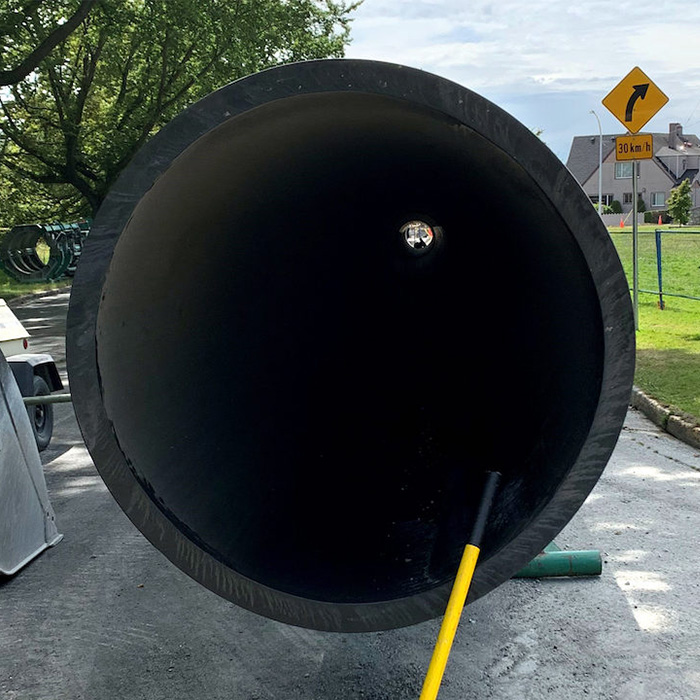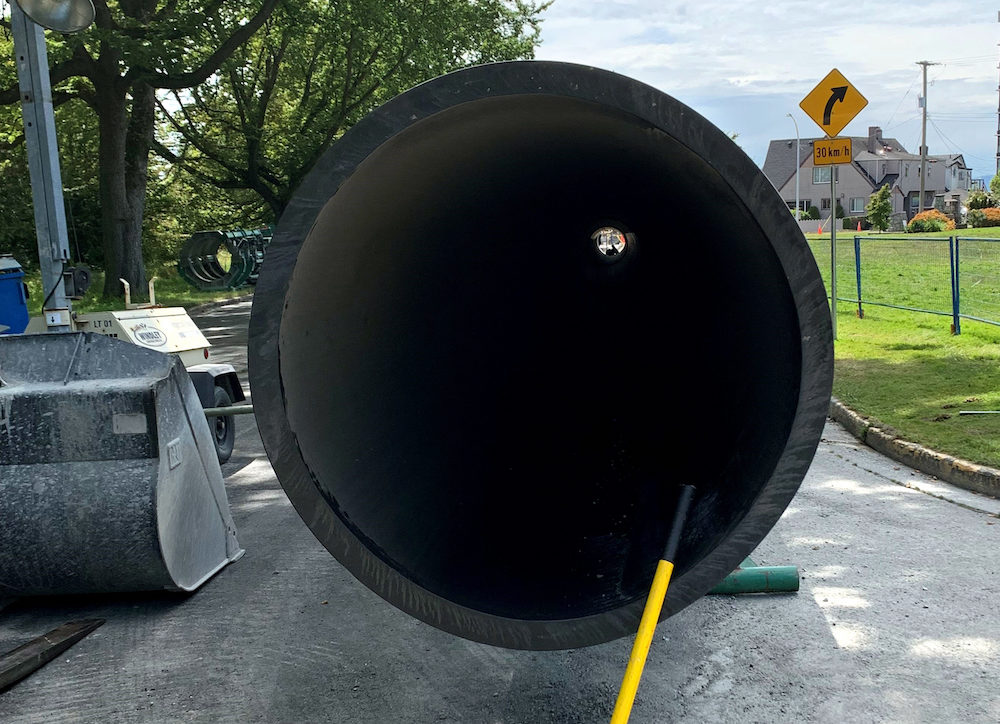Guide to Standard Dimension Ratio (SDR)

# Guide to Standard Dimension Ratio (SDR)Guide to Standard Dimension Ratio (SDR)The standard dimension ratio (SDR)—also referred to as the standard dimensional ratio—is a measurement and method often used by pipe manufacturers to rate the working pressure capacity of a pipe. It is calculated by determining the relationship of the pipe’s outside diameter to its minimum wall thickness. The higher the calculated SDR, the lower the amount of pressure it can handle since the pipe minimum wall thickness is reduced in proportion to the outside diameter remaining the same. The lower the calculated SDR, the higher the amount of pressure it can handle since the wall thickness is thicker in proportion to the pipe diameter. As an example, SDR17 has a thinner wall and lower pressure class than an SDR 11. Knowing and understanding this value is essential for many applications, including, but not limited to, the following:

• When calculating the internal pressure inside of a pipe system

• When inserting objects into a pipe system such as ID stiffeners for compression fittings

• When determining the tensile strength of a pipe under high stress

• When assessing the compressive strength of a pipeline undergoing burial